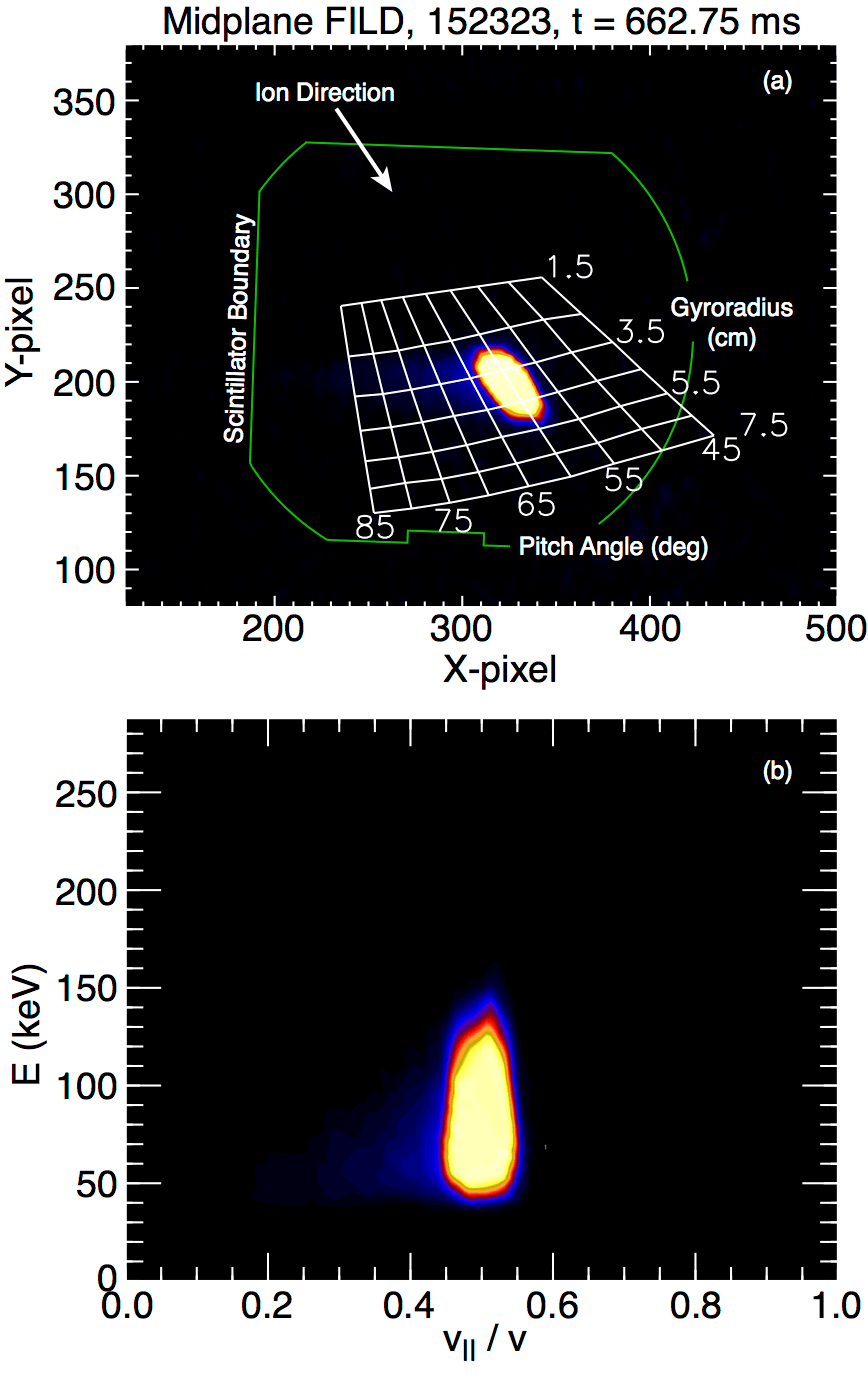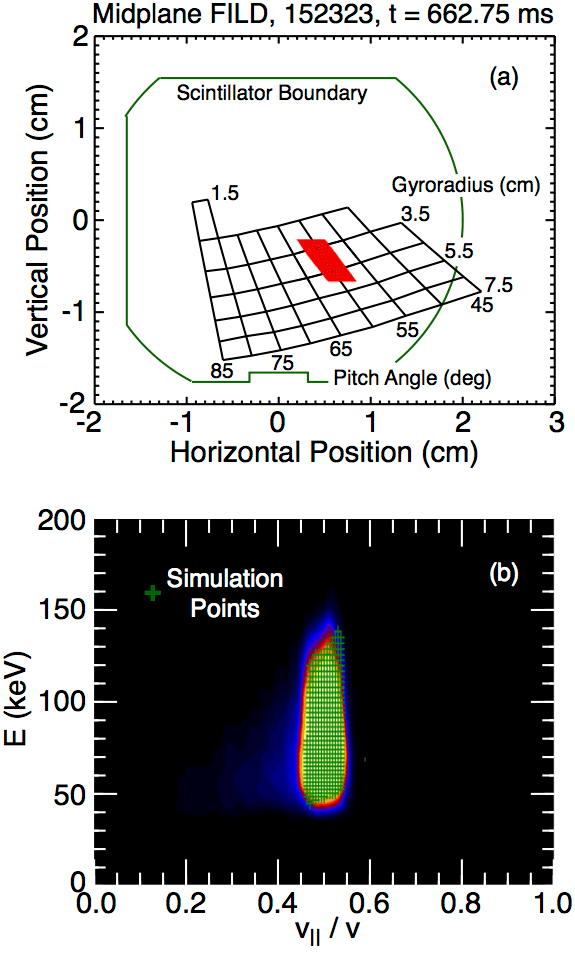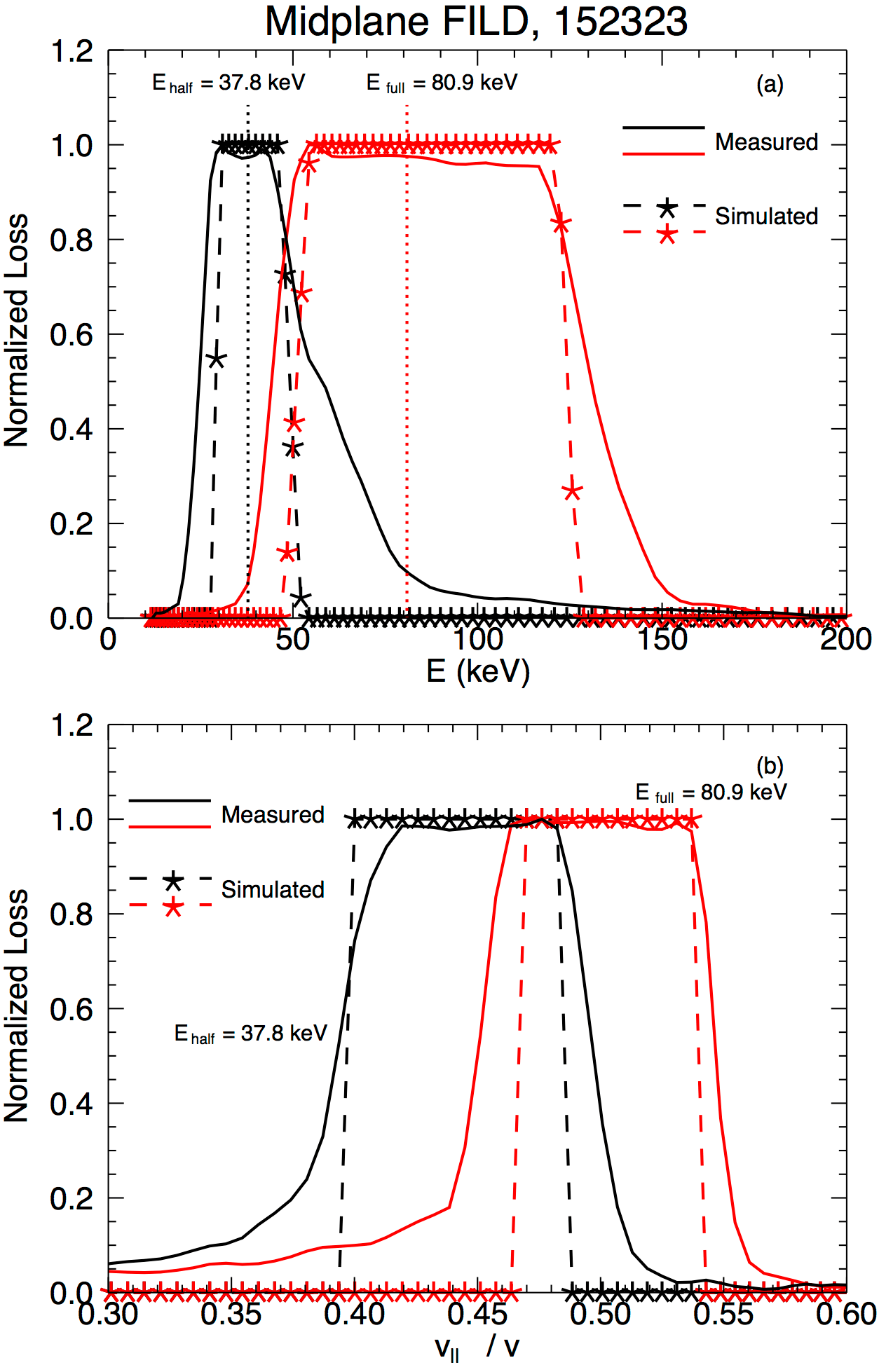# Mapping and Uncertainty Analysis of Energy and Pitch Angle Phase Space in the DIII-D Fast Ion Loss Detector

The following work is a technical diagnostic paper concerning a method for calculating the uncertainty in a probe that measures energetic ion losses in the DIII-D tokamak. The fast ion loss detector (FILD) measures the pitch angle and the energy of these ions that hit it at the outer wall. That measurement is incredibly valuable for studies of how these high energy ions move throughout the tokamak because the energy and pitch angle is a comprehensive data set for identifying the trajectory of the ions. As such, the uncertainty in this measurement should be considered when the data is used for modeling of ion transport.

It’s also worthwhile to note that the co-author R. Pipes was a summer intern at DIII-D in 2013 and his project work contributed to this result. It can be difficult for an undergraduate student to be so productive in only nine weeks, so being a co-author on a paper is an achievement.

This paper appears in Review of Scientific Instruments as,
D.C. Pace, R. Pipes, R.K. Fisher, and M.A. Van Zeeland, Rev. Sci. Instrum. 85, 11D841 (2014).

## Mapping and Uncertainty Analysis of Energy and Pitch Angle Phase Space in the DIII-D Fast Ion Loss Detector

### Abstract

New phase space mapping and uncertainty analysis of energetic ion loss data in the DIII-D tokamak provides experimental results that serve as valuable constraints in first-principles simulations of energetic ion transport. Beam ion losses are measured by the fast ion loss detector (FILD) diagnostic system consisting of two magnetic spectrometers placed independently along the outer wall. Monte Carlo simulations of mono-energetic and single-pitch ions reaching the FILDs are used to determine the expected uncertainty in the measurements. Modeling shows that the variation in gyrophase of 80 keV beam ions at the FILD aperture can produce an apparent measured energy signature spanning across 50-140 keV. These calculations compare favorably with experiments in which neutral beam prompt loss provides a well known energy and pitch distribution.

While the confinement of fusion-α particles in a magnetically confined DT-reactor is essential to maintain a burning state, present tokamaks make excellent use of lost energetic ions to study the effects of MHD and other performance limiting phenomena. Fast ion loss detectors (FILDs) are magnetic spectrometers (inspired by the original diagnostic on TFTR) that use the tokamak’s magnetic field to direct energetic ions into a scintillator plate.[2-4] The striking position of the ion along the face of the scintillator is a function of its energy and pitch angle with respect to the local magnetic field. This measurement fully describes the lost ion and allows for the calculation of its orbit trajectory on approach to the FILD.

The design of the FILD installed on the ASDEX Upgrade tokamak  was used in the implementation of two FILDs at DIII-D. [3,4] Resulting measurements of energetic ion losses have since informed efforts to understand many tokamak instabilities and processes including fishbones, Alfvén eigenmodes, energetic particle-induced geodesic acoustic modes, and applied magnetic perturbations. Comprehensive understanding of such plasma behavior requires theoretical models and simulations that reproduce the observations. Full orbit codes such as SPIRAL have emerged to simulate tokamak experiments while including relevant interactions between the ions and plasma fluctuations (which may be modeled by separate codes to determine an eigenfunction or fluctuation amplitude).FIG. 1: (a) Drawing of the midplane FILD indicating dimensions of the collimating aperture (orange component). (b) Cross-section of the midplane FILD including an example ion orbit (red dashed line) passing through the collimator.

As the fidelity of simulations continues to improve, it falls to the experiments to reduce uncertainty and provide constraints that determine the validity of the theoretical models. With this motivation, a study of the uncertainty associated with energy and pitch angle measurements of the DIII-D FILD is undertaken. Through this process a mechanism by which FILD data is consistently translated in energy/pitch space is developed.

Figure 1 illustrates the discrete nature of the FILD collimator. The collimator piece is shown in orange and an example ion trajectory is drawn by the red dashed line in Fig. 1(b). Figure 2 shows the analysis path of FILD measurements. Figure 2(a) displays a false color camera frame. This is taken from a FILD calibration shot (152323 with$B_t = 2.1$ T) in which each of DIII-D’s eight neutral beams are injected individually during plasma current ramps to create prompt loss spots on the scintillators. The beams inject at distinct energy levels (full, one-half, and one-third) and the short transit time of prompt loss beam ions is confirmed with fast-sampled photomultipliers (PMTs). This allows a beam ion orbit with known energy and pitch angle to validate the strike map  overlaid in white in Fig. 2(a). No color scale is provided for this example because the prompt loss is large enough to saturate the camera. This map is calculated with a modified version of NLSDETSIM. EFIT  magnetic reconstructions are used to calculate the magnetic field at the FILD and then helixes are calculated beginning at various positions within the collimator. The strike map grid points represent the centroid of strike locations for each modeled ion trajectory. Gyroradius labels actually represent (through historical convention) isoenergy lines, i.e., the gyroradius that would result for an ion of fixed energy and a$90^\circ$ pitch angle. The strike map is aligned based on the camera view as adjusted when backlighting the scintillator. A final qualitative check is performed by noting that the elongated prompt loss stripe is parallel to a pitch angle line as it must be based on the geometry of incoming ions.FIG. 2: (a) Camera frame from the midplane FILD with an overlay of the strike map, scintillator outline, and approaching ion direction shown. (b) Resulting energy/pitch phase space grid obtained by converting the mapped frame of panel (a).

The camera data as shown in Fig. 2(a) provides the best resolution of energy and pitch angle (PMT data is collected through discrete fiber optic views that sample a wider region of the scintillator than any single camera pixel). Simulations need FILD data in terms of energy, however, so this camera output must be analyzed and converted from its gyroradius representation. Such a result is shown in Fig. 2(b) where the camera data has been converted to a more standard energy/pitch grid. Pitch is represented by the ratio of the ion’s parallel to total velocity with respect to the magnetic field,$v_\parallel/v$, and the pitch angle is$\chi = \cos^{-1}(v_\parallel/v)$. To complete this conversion, the camera frame is triangulated based on the strike map. The magnetic field amplitude at the FILD is known in order to calculate the strike map, and that information is used to convert the gyroradius points into total ion energy. The triangulation is only performed inside of the mapped region.

In this prompt loss scenario, the measured loss region in Fig. 2 represents a single energy and pitch angle of loss. The incident ions feature energy E = 80.86 keV (set by the injecting beam) and pitch angle$\chi = 60^\circ \;(v_\parallel/v = 0.5$ is known from the calibration shot analysis). Any observed spread in the loss region is due to the combined effects of the spatially extended neutral beam injection footprint and the discrete nature of the FILD aperture.

Figure 3 shows the method of the uncertainty analysis and demonstrates that the observed widths (in gyroradius and pitch angle) are consistent with the spread due to the geometry of the collimating aperture. In Fig. 3(a) a strike map is shown in real space with the scintillator boundary indicated by the solid green line. Red points indicate the strike positions of individual simulated ions with a gyroradius of$r_L = 3.974$ cm (equivalent to the known E = 80.86 keV). These ions strike at different positions across the scintillator because they reach the collimator at different values of gyrophase. Physically, this is simulated by beginning the ions at different positions within the collimator. Those trajectories that avoid hitting a physical structure within the FILD are traced to their impact point on the scintillator surface. Impact points are converted to energy/pitch phase space using the same method as applied to the camera data and the result is shown in Fig. 3(b). The simulated mono-energetic and single-pitch ions are overlaid as green$+$-symbols on the result from Fig. 2(b). The result is a nearly perfect match between the measured and simulated losses. The agreement provides an additional verification of the prompt loss calibration method.FIG. 3: (a) Strike map calculated in real space for the midplane FILD. The red portion represents the full set of individual strikes from ions with gyroradius$r_L = 3.974$ cm (E = 80.86 keV) and pitch angle$\chi = 60^\circ \;(v_\parallel/v = 0.5)$. (b) The individual strikes from panel (a) are overlaid on the energy/pitch phase space contour from Fig. 2(b).

An example analysis comparing the energy and pitch resolution for two different prompt loss cases in shot 152323 is shown in Fig. 4. The red data represent the full energy prompt loss from Figs. 2 and 3 and the black traces represent the$t = 1920$ ms beam pulse from that shot during which a one-half beam energy ($E_{half} = 37.8$ keV for that beam) prompt loss was observed. The *-symbol traces with dashed lines represent the simulation. Figure 4(a) plots the results as a function of energy taken through the central pitch of the measured spots. The energy ranges spanned by these mono-energetic loss spots are$\Delta E/E \approx 1$ for the full energy case and$\Delta E/E \approx 0.5$ for the half-energy case. Improved energy resolution at lower values of$E$ occurs because of the relationship between gyro-orbit curvature and the radial width of the collimator. Considering Fig. 1(b) with a fixed “Collimator Width” and depth, the gyrophase selection range narrows as the gyroradius becomes smaller. Conversely, ions with larger gyroradii have a wider range of gyrophases that will pass through the collimator, i.e., more of the incident trajectories are nearly vertical as they pass through the relatively small collimator. One benefit of this dependence is that a FILD collimator can be designed to achieve any theoretical energy resolution regardless of the tokamak magnetic field strength or physical size (though signal levels will eventually limit the achievable energy resolution). The$\Delta E/E$ values in the present case are comparable to the identified actual ion energy, suggesting that simulations of the incident ions are necessary to have confidence in the measurement. Figure 4(b) provides an example of the pitch range observed for these prompt loss cases. The full energy case centers on$v_\parallel/v = 0.5$ while the half-energy case centers on$v_\parallel/v = 0.4$. Pitch ranges in these cases are$\Delta \chi = 5^\circ$.

The agreement between simulated and measured FILD signals in these cases demonstrates that improved determination of energy and pitch measurements is possible. This capability will be applied in a design study identifying optimal adjustments to the collimators in order to improve the energy resolution of FILDs.FIG. 4: (a) Normalized loss profiles through the center of the loss spots for a full energy ($E_{full} = 80.9$ keV) and half energy ($E_{half} = 37.8$ keV) prompt loss scenario. (b) Normalized loss profiles through the specified beam energies for the scenarios of panel (a). Simulated profiles are given by the -*- traces.

This work was supported in part by the US Department of Energy under DE-FC02-04ER54698 and the National Undergraduate Fellowship in Fusion Science and Engineering. DIII-D data shown in this paper can be obtained in digital format by following the links at https://fusion.gat.com/global/D3D_DMP. In addition, the authors are grateful to A. Chavez for providing the FILD CAD drawings and D. Darrow for providing the NLSDETSIM code and assisting in its modification.

1 S. J. Zweben, Rev. Sci. Instrum. 60, 576 (1989).

2 M. García-Muñoz, H.-U. Fahrbach, H. Zohm, and the ASDEX Upgrade Team, Rev. Sci. Instrum. 80, 053503 (2009).

3 R. K. Fisher, D. C. Pace, M. García-Muñoz, W. W. Heidbrink, C. M. Muscatello, M. A. Van Zeeland, and Y. B. Zhu, Rev. Sci. Instrum. 81, 10D307 4 (2010).

4. X. Chen, R. K. Fisher, D. C. Pace, M. García-Muñoz, J. A. Chavez, W. W. Heidbrink, and M. A. V. Zeeland, Rev. Sci. Instrum. 83, 10D707 (2012).

5 W. W. Heidbrink et al., Plasma Phys. Control. Fusion 53, 085028 (2011).

6 M. A. Van Zeeland et al., Phys. Plasmas 18, 056114 (2011).

7 R. Fisher, D. Pace, G. Kramer, M. V. Zeeland, R. Nazikian, W. Heidbrink, and M. García-Muñoz, Nucl. Fusion 52, 123015 (2012).

8 M. A. Van Zeeland et al., Plasma Phys. Control. Fusion 56, 015009 (2014).

9 G. J. Kramer, R. V. Budny, A. Bortolon, E. D. Fredrickson, G. Y. Fu, W.W. Heidbrink, R. Nazikian, E. Valeo, and M. A. Van Zeeland, Plasma Phys. 10 Control. Fusion 55, 025013 (2013).

10 D. C. Pace, R. K. Fisher, M. García-Muñoz, D. S. Darrow, W. W. Heidbrink, C. M. Muscatello, R. Nazikian, M. A. Van Zeeland, and Y. B. Zhu, Rev. Sci. Instrum. 81, 10D305 (2010).

11 S. Zweben, R. Boivin, M. Diesso, S. Hayes, H. Hendel, H. Park, and J. Strachan, Nucl. Fusion 30, 1551 (1990).

12 L. L. Lao, H. E. S. John, Q. Peng, J. R. Ferron, E. J. Strait, T. S. Taylor, W. H. Meyer, C. Zhang, and K. I. You, Fusion Sci. Technol. 48, 968 (2005).